# 1743 Finding Ways to Transform My Heart

### Today’s Puzzle:

An equation of a unit circle centered at the origin is x² + y² = 1.

If we change just the “y” part of that equation, we can get a lovely heart just in time for Valentine’s Day. Try it yourself by typing the equations into Desmos.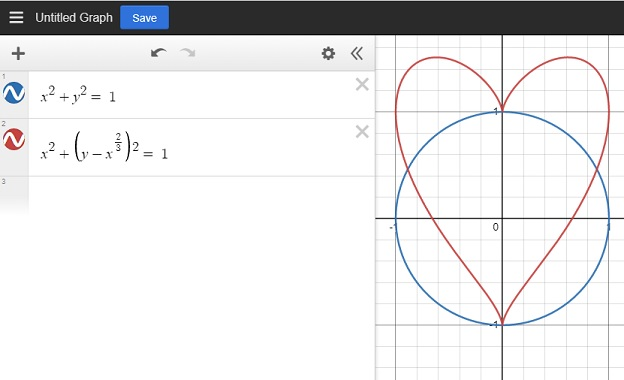There are other mathematical equations for a heart, but this is the one I’m exploring in this post.

I was puzzled over how I could transform that heart. Can I make it bigger, or dilate it? Can I slide it away from the origin or translate it? Can I rotate it? Can I reflect it across the x or y-axis?

These are questions I’d like you to explore as well.

### Heart Dilation:

In this first graphic, I was able to make my heart bigger. What kind of math let me do that? Also, how did I color the inside of some of the hearts? Look at the equations next to the heart and try to figure it out. The concentric hearts are evenly spaced. Do you recognize a pattern in the numbers that made that happen?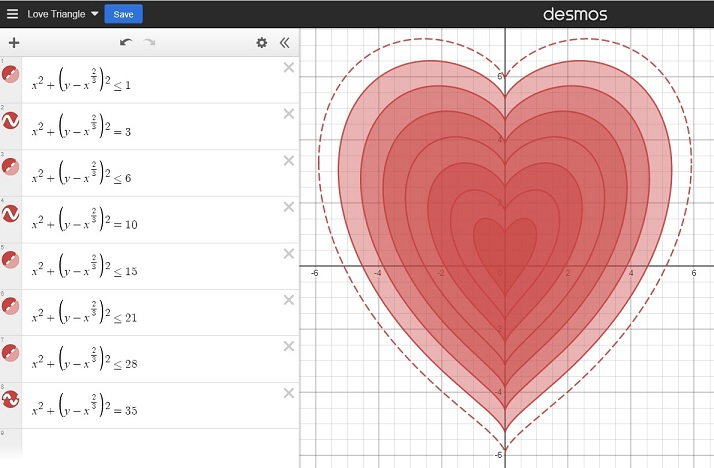### Heart Slide (Translation):

If we changed the center of a circle to (a, -b) instead of the origin, we would slide the whole circle. Here’s how we change the equation of the circle to give it a new center:
(x-a)²+(y+b)² = 1.

Similarly, in the next graphic, I was able to slide my heart away from the origin. How did I do that? Look at the equations to see how.### Heart Rotation:

A circle looks the same no matter how it is rotated, but the same isn’t true for a heart. Look at the equations below. How was I able to rotate my heart around the origin?### Heart Reflection:

Since a heart is symmetric, its reflection across the x-axis doesn’t look that interesting to me. Instead, I created a double heart that I reflected across both the x-axis and the y-axis: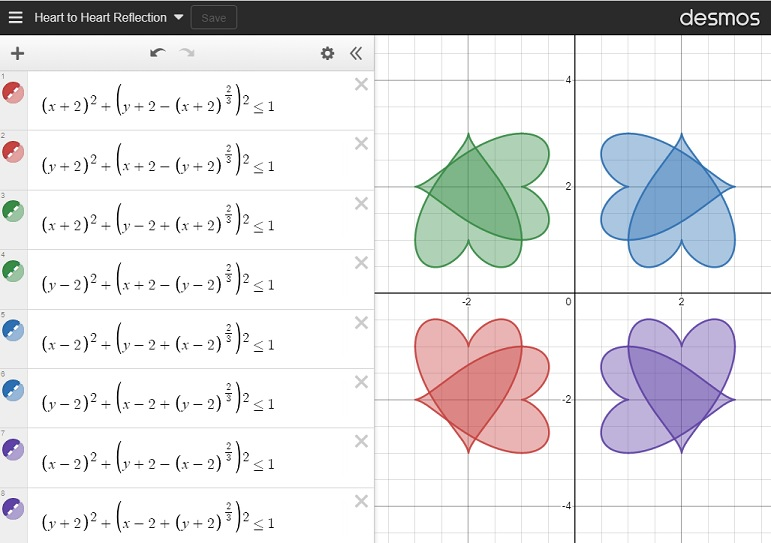### Just for Fun:

Next, I was curious about what would happen if I changed the exponents on the outside of the parenthesis, so I changed a 2 from my original equation to an 8 in a couple of different places as I moved the heart from left to right. How did changing the exponent affect my heart? I found that as long as the exponent stays even, it still looks a little like a heart.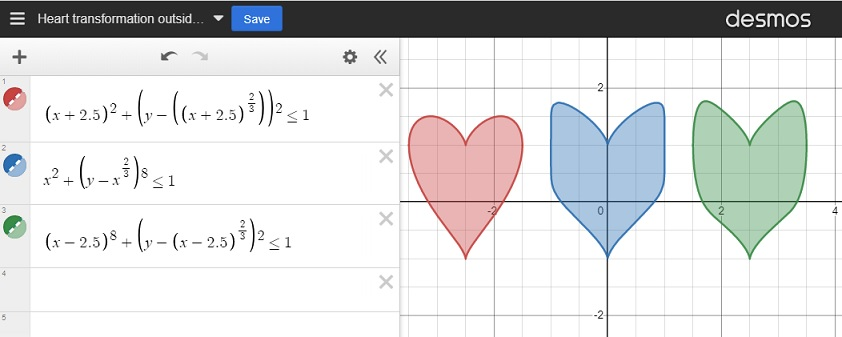I was also curious about what would happen to my heart if I changed the “2/3” to a different fraction. I used fractions less than one as well as fractions greater than one. For many of my fractions, I used the post number, 1743, as the denominator. As long as the numerator was even and the denominator was odd, the graph still looked mostly like a heart. However, the closer the fraction was to zero, the more it looked like a circle.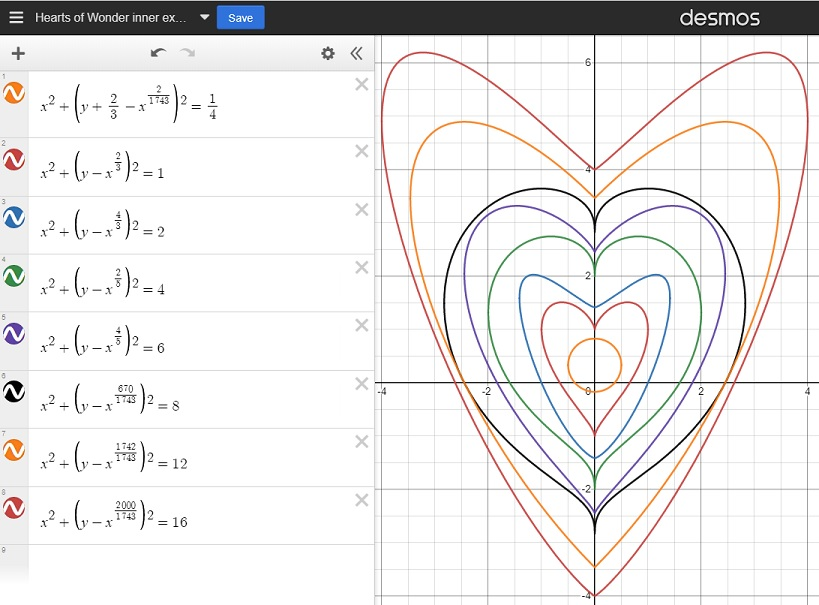Finally, I created this lovely flower using some of what I learned by making these transformations:And for just a little bit more fun, I created a simple but chaotic-looking animation that I’ve titled Hearts in Motion. Enjoy!

I had so much fun exploring this heart in Desmos. Thank you for allowing me to share my excitement with you. Here’s a different Desmos heart created by a reader and shared with me. Click on it to make the heart beat:

### Factors of 1743:

• 1743 is a composite number.
• Prime factorization: 1743 = 3 × 7 × 83.
• 1743 has no exponents greater than 1 in its prime factorization, so √1743 cannot be simplified.
• The exponents in the prime factorization are 1, 1, and 1. Adding one to each exponent and multiplying we get (1 + 1)(1 + 1)(1 + 1) = 2 × 2 × 2 = 8. Therefore 1743 has exactly 8 factors.
• The factors of 1743 are outlined with their factor pair partners in the graphic below.### More About the Number 1743:

1743 is the difference of two squares in four different ways:
872² – 871² = 1743,
292² – 289² = 1743,
128² – 121² = 1743, and
52² – 31² = 1743.

# 1314 Desmos Art

A teacher at my school had his students graph some polynomials and their inverses. I got to help some of his students with their graphs. After seeing the beautiful symmetry of the graphs together, I excitedly exclaimed to a few of the students, “Isn’t this a cool assignment?”

During my lunch, I put one of the graphs, its inverse, and some of their translations on Desmos and made a simple but lovely piece of art in the process.

Before I was done, I showed it to a couple of students. One of them asked, “Are you saying that math can create art?” I loved replying, “Yes, it can!” Now that student wants to create some works of art, too. It was a privilege to show her how to use Desmos.

These are the inequalities I used to make my work of art:

MANY teachers have figured out that students could learn a lot about functions and their graphs by using Desmos to create drawings, pictures, or artwork. For example, look at this tweet and link shared by Chris Bolognese:

Now I’ll share some facts about the number 1314:

• 1314is a composite number.
• Primefactorization: 1314= 2 × 3 × 3 × 73,which can be written 1314 = 2 × 3² × 73
• The exponents inthe prime factorization are 1, 2, and 1. Adding one to each and multiplying weget (1 + 1)(2 + 1)(1 + 1) = 2 × 3 × 2 = 12. Therefore 1314has exactly 12 factors.
• Factors of 1314:1, 2, 3, 6, 9, 18, 73, 146, 219, 438, 657, 1314
• Factor pairs: 1314= 1 × 1314,2 × 657, 3 × 438, 6 × 219, 9 × 146, or 18 × 73
• Taking the factorpair with the largest square number factor, we get √1314= (√9)(√146) = 3√146 ≈ 36.24914

1314 is the sum of two squares:
33² + 15² = 1314

1314 is the hypotenuse of a Pythagorean triple:
864-990-1314 which is 18 times (48-55-73) and
can also be calculated from 33² – 15², 2(33)(15), 33² + 15²